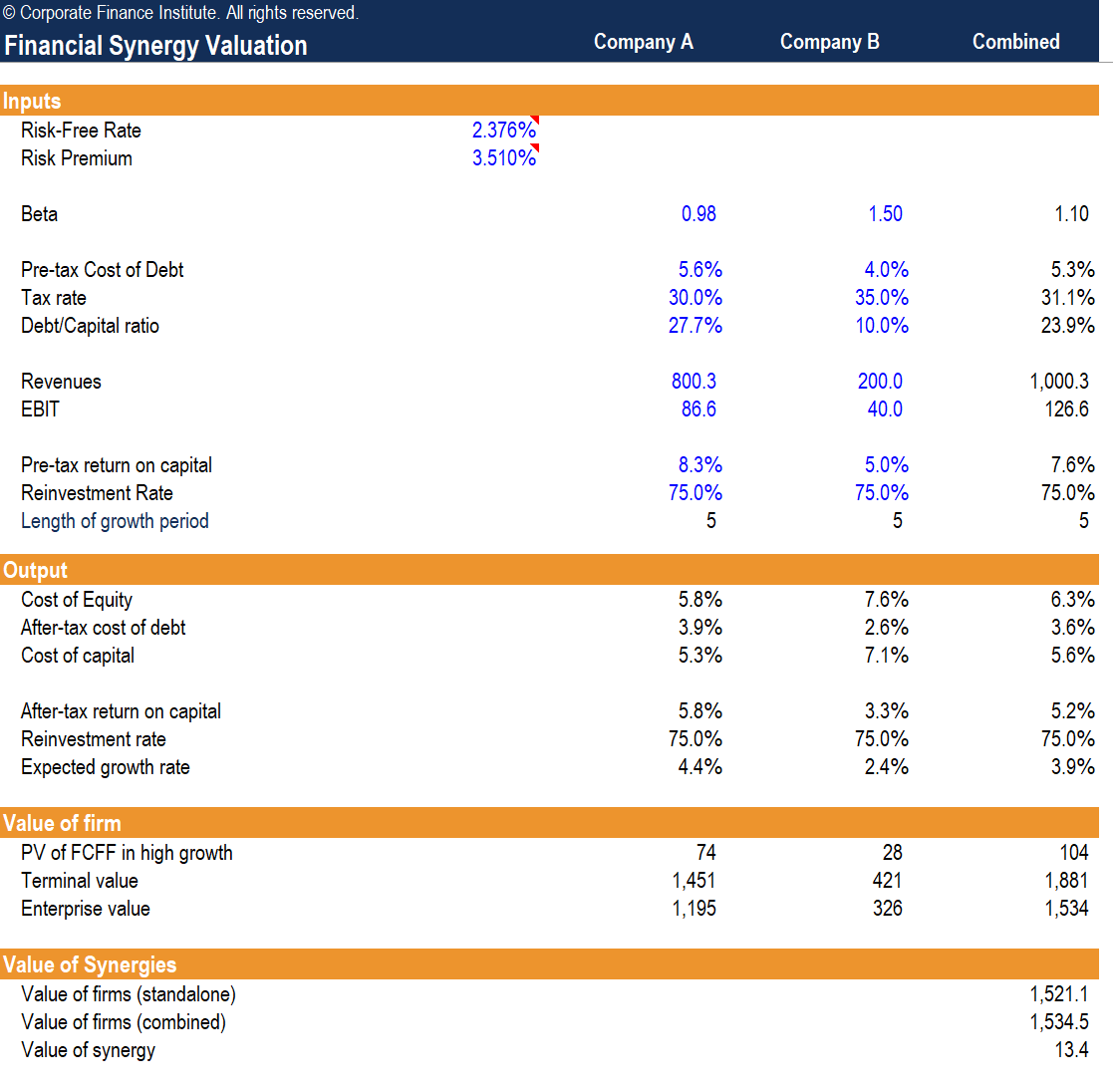# Financial Synergy Valuation

How to value synergies from a merger or acquisition

## What is Synergy Valuation?

When a company acquires another business, it is often justified by the argument that the investment will create synergies. The primary source of synergy in an acquisition is in the presumption that the target firm controls a specialized resource that becomes more valuable if combined with the acquiring firm’s resources. There are two main types, operating synergy and financial synergy, and this guide will focus on the latter.### Types of Synergies

Synergy can be categorized into two forms: operating synergy and financial synergy.

#### 1. Operating synergy

Operating synergies create strategic advantages that result in higher returns on investment and the ability to make more investments and more sustainable excess returns over time. Furthermore, operating synergies can result in economies of scale, allowing the acquiring company to save costs in current operations, whether it be through bulk trade discounts from increased buyer power, or cost savings by eliminating redundant business lines.

Types of operating synergies to value include:

• Horizontal Integration: Economies of scale, which reduce costs, or from increased market power, which increases profit margins and sales.
• Vertical Integration: Cost savings from controlling the value chain more comprehensively.
• Functional Integration:  When a firm with strengths in one functional area acquires another firm with strengths in a different functional area, the potential synergy gains arise from specialization in each respective functional area.

#### 2. Financial synergy

Financial synergies refer to an acquisition that creates tax benefits, increased debt capacity and diversification benefits. In terms of tax benefits, an acquirer may enjoy lower taxes on earnings due to higher depreciation claims or combined operating loss carryforwards. Second, a larger company may be able to incur more debt, reducing its overall cost of capital. And lastly, diversification may reduce the cost of equity, especially if the target is a private or closely held firm.

### Using the Financial Synergy Valuation Worksheet

The Synergy Valuation Excel Model enables you – with the beta, pre-tax cost of debt, tax rate, debt to capital ratio, revenues, operating income (EBIT), pre-tax return on capital, reinvestment rate and length of growth period – to compute the value of the global synergy in a merger.### Financial Synergy Valuation Template

#### Steps to value financial synergies

1. Enter the assumptions for the risk-free rate and the risk premium. The worksheet default contains sources for the base assumptions, using the US 10-year Treasury rate as a proxy for the risk-free rate and the US risk premium from market-risk-premia.com
2. Enter the inputs for both the target and acquiring companies. The model will be driven by the variables described above.
3. The combined firm’s financial metrics are calculated as follows:
• Beta:
• Estimate the unlevered betas for both the target and acquiring companies
• Estimate the unlevered beta for the combined firm by taking an enterprise-value weighted average
• Estimate the levered beta for the combined firm using the debt to equity ratio of the combined firm
• Pre-tax cost of debt: EV weighted-average pre-tax cost of debt of both firms
• Tax rate: EV weighted-average tax rate of both firms
• Debt to capital ratio: EV weighted-average debt to capital ratio of both firms
• Revenue: Sum of both firms’ revenues
• EBIT: Sum of both firms’ EBIT
• Pre-tax return on capital: EV weighted-average pre-tax return on capital of both firms
• Reinvestment rate: EV weighted-average reinvestment rate of both firms
1. The output section computes the base assumptions that will be used to value both firms standalone and combined:
• The cost of capital uses the WACC formula and will be used to discount future cash flows
• The expected growth rate estimated by the product of the after-tax return on capital and the assumed reinvestment rate.
2. The valuation section computes the enterprise value of both firms standalone and combined:
• The present value of free cash flows to both firms standalone and combined is calculated by taking NOPAT (EBIT * 1 – tax rate) and multiplying it by the reinvestment rate. The present value of a growing annuity formula is applied, grown using the expected growth rate and discounted by the cost of capital computed above.
• The terminal value of both firms standalone and combined is calculated by applying the growing perpetuity formula to the terminal year’s free cash flow.
• The enterprise value is the sum of both firms standalone and combined present values of free cash flows and the present value of terminal values.
3. The value of financial synergies is determined by taking the difference in enterprise values of both firms standalone and combined.

### Key Takeaway

The valuation method described above allows us to determine the financial synergies of the combined company. From the analysis, the primary driver of financial synergies is the benefit implied by combining the cost of financing of both companies. The value of financial synergies can be analyzed further by finding the proportion of the value of synergy attributable to the specific sources of synergies discussed above.